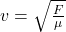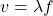## A “mechanical wave” occurs in a physical medium because of some restoring force acting on the medium, and can be described by an amplitude,

Question

A “mechanical wave” occurs in a physical medium because of some restoring force acting on the medium, and can be described by an amplitude, an oscillation frequency, and an amplitude.

You can easily decrease the wavelength of a mechanical wave by half by doubling the frequency ONLY if which of the following is true? Select all that apply

(A) The ratio between the restoring force and the density of the medium remain constant
(B) The amplitude is doubled
(C) The amplitude is reduced by 1/2
(D) The same medium is used at the same temperature

in progress 0
4 months 2021-09-04T08:13:57+00:00 1 Answers 72 views 0

## Answers ( )

(A) The ratio between the restoring force and the density of the medium remain constant

Explanation:

The ratio between the restoring force and the density of the medium is equal to the square of the velocity of the wave.The general formula that relates the displacement and velocity (x = vt) can be written in wave mechanics such thatwhere f is the frequency, λ is the wavelength, and v is the velocity of the wave.

According to this equation, in order to halve the wavelength by doubling the frequency, the velocity should be constant. Therefore, the correct answer is (A).